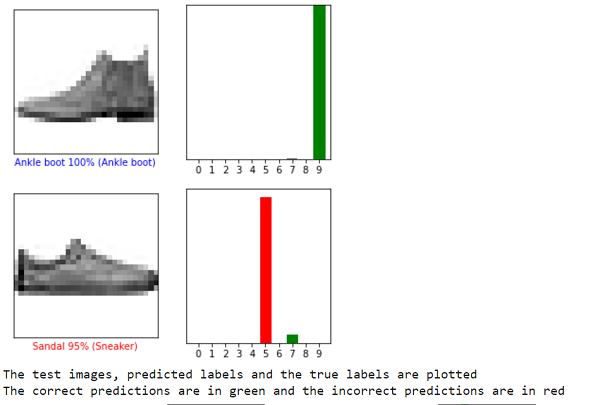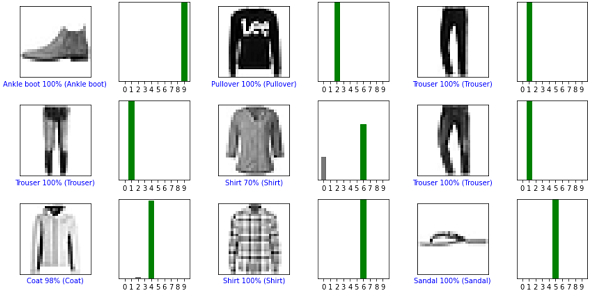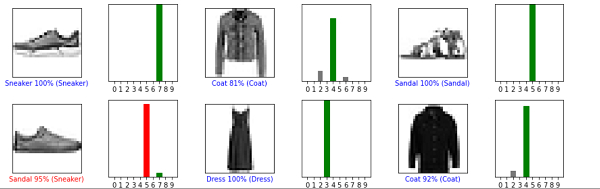# How can TensorFlow be used to verify the predictions for Fashion MNIST in Python?

Tensorflow is a machine learning framework that is provided by Google. It is an open−source framework used in conjunction with Python to implement algorithms, deep learning applications and much more. It is used in research and for production purposes.

The ‘tensorflow’ package can be installed on Windows using the below line of code −

pip install tensorflow

The ‘Fashion MNIST’ dataset contains images of clothing of different kinds. It contains grayscale images of more than 70 thousand clothes that belong to 10 different categories. These images are of low resolution (28 x 28 pixels).

We are using the Google Colaboratory to run the below code. Google Colab or Colaboratory helps run Python code over the browser and requires zero configuration and free access to GPUs (Graphical Processing Units). Colaboratory has been built on top of Jupyter Notebook.

Following is the code snippet to verify the predictions for Fashion MNIST in Python −

## Example

i = 0
plt.figure(figsize=(6,3))
plt.subplot(1,2,1)
plot_image(i, predictions[i], test_labels, test_images)
plt.subplot(1,2,2)
plot_value_array(i, predictions[i],  test_labels)
plt.show()

i = 12
plt.figure(figsize=(6,3))
plt.subplot(1,2,1)
plot_image(i, predictions[i], test_labels, test_images)
plt.subplot(1,2,2)
plot_value_array(i, predictions[i],  test_labels)
plt.show()

num_rows = 5
num_cols = 3
print("The test images, predicted labels and the true labels are plotted")
print("The correct predictions are in green and the incorrect predictions are in red")
num_images = num_rows*num_cols
plt.figure(figsize=(2*2*num_cols, 2*num_rows))
for i in range(num_images):
plt.subplot(num_rows, 2*num_cols, 2*i+1)
plot_image(i, predictions[i], test_labels, test_images)
plt.subplot(num_rows, 2*num_cols, 2*i+2)
plot_value_array(i, predictions[i], test_labels)
plt.tight_layout()
plt.show()

## Output## Explanation

• Once the model has been trained, it can be used to make predictions on other images.

• The predictions are made on an image, and the prediction array is displayed.

• The correctly predicted labels are in green and the incorrectly predicted ones are in red.

• The number indicates the percentage value for the predicted label.

• It tells how accurately the model suggests that the label it has predicted is the actual label for the image.